TitleCollege Algebra
Answer/Discussion to Practice Problems
Tutorial 48: Practice Test on Tutorials 42 - 47

 Problems 1a - 1b: Graph the following functions.

1a.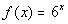Answer:

 x(x, y) -2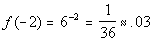(-2, .03) -1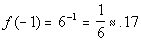(-1, .17) 0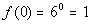(0, 1) 1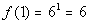(1, 6) 2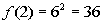(2, 36)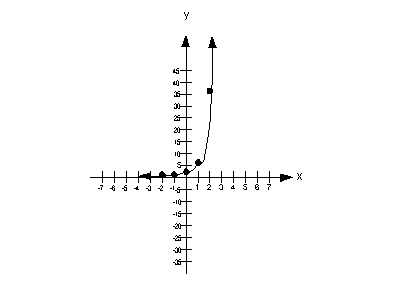1b.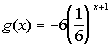Answer:

 x(x, y) -2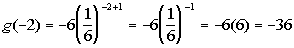(-2, -36) -1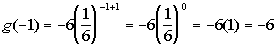(-1, -6) 0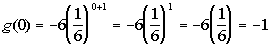(0, -1) 1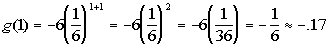(1, -.17) 2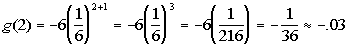(2, -.03)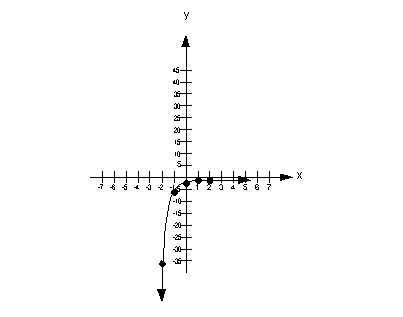Problem 2a:   Find the 1) compound amount AND 2) the compound interest for the given investment and rate.

2a. \$25000 for 21 years at an annual rate of 3.25% compounded quarterly.Answer: P = 25000 r = 3.25% = .0325 t = 21 n = quarterly = 4 times a year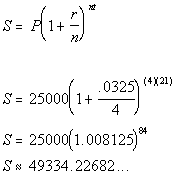Compound Amount: \$49334.23 Compound Interest: \$24334.23

 Problem 3a: Find the accumulated value for the given investment and  rate.

3a. \$4525 that is compounded continuously for 12 years at an interest rate of 9 ½ %.Answer: P = 4525 r = 9.5% = .095  t = 12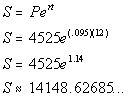So the accumulated or compound AMOUNT would be \$14148.63.

 Problems 4a - 4b: Express the given logarithmic equation exponentially.

4a.Answer: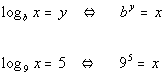4b.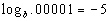Answer: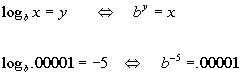Problems 5a - 5b:  Express the given exponential equation in a logarithmic form.

5a.Answer: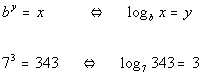5b.Answer: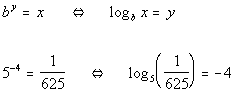Problems 6a - 6b:   Evaluate the given log function without using a calculator.

6a.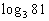Answer: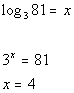6b.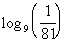Answer: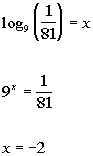Problems 7a - 7b: Graph the following functions.

7a.Answer: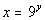y (x, y)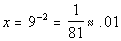-2 (.01, -2)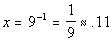-1 (.11, -1)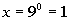0 (1, 0)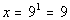1 (9, 1)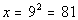2 (81, 2)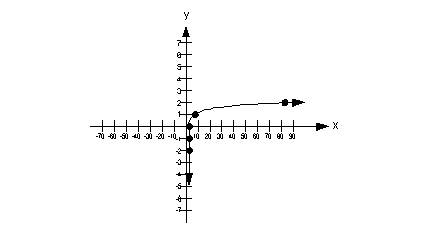7b.Answer: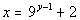y (x, y)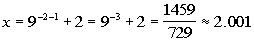-2 (2.001, -2)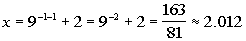-1 (2.012, -1)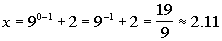0 (2.11, 0)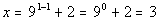1 (3, 1)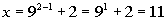2 (11, 2)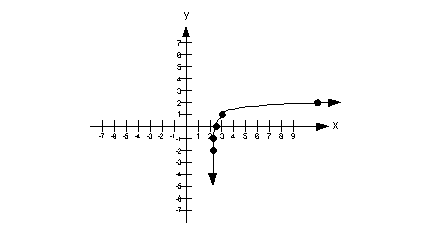Problems 8a - 8b:   Evaluate the given expression without the use of a calculator.

8a.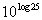Answer: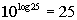8b.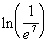Answer: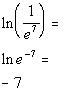Problems 9a - 9b:  Expand each logarithmic expression as much as possible.  Evaluate without a calculator where possible.

9a.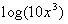Answer: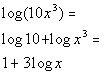9b.Answer: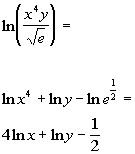Problems 10a - 10b:  Condense each logarithmic expression into one  logarithmic expression.  Evaluate without a calculator  where possible.

10a.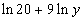Answer: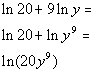10b.Answer: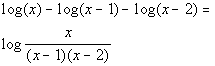Problems 11a - 11c:    Solve the given exponential equation.  Round your answer to two decimal places.

11a.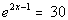Answer: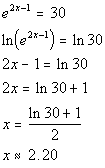11b.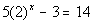Answer: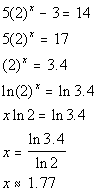11c.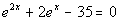Answer: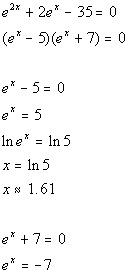Since e raised to a power cannot equal a negative number, there is only one solution, x is approx 1.61.

 Problems 12a - 12b:   Solve the logarithmic equation.  Round your answer to two decimal places.

12a.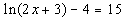Answer: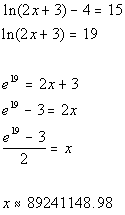12b.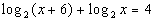Answer: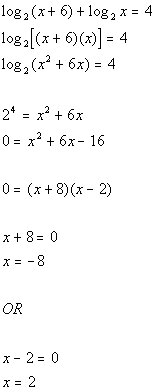x = 2 is the only solution.

Last revised on March 25, 2011 by Kim Seward.
All contents copyright (C) 2002 - 2011, WTAMU and Kim Seward. All rights reserved.